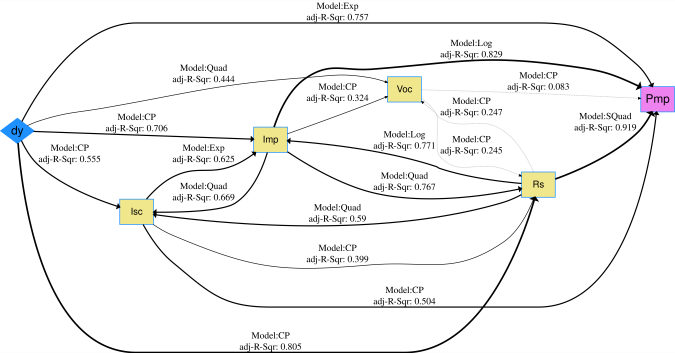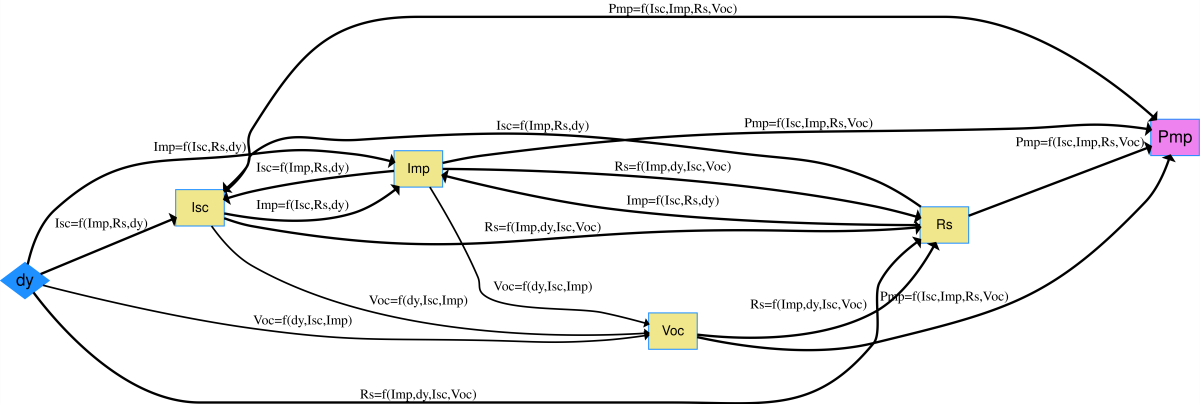# Current-Voltage(I-V) Features of Photovoltaic Modules

## Data Description

The dataset consists of current-voltage($$I-V$$) features obtained by $$I-V$$ feature extraction from $$I-V$$ curves of photovoltaic (PV) modules. The features have been extracted using the ddiv package algorithm for brand A PV modules under damp heat indoor accelerated exposures for up to 3000 hours. The I-V curves were measured in a step-wise manner after every 500h of exposure time, and provided by the SunEdison company. The I-V features include max power ($$P_{mp}$$), short circuit current ($$I_{sc}$$), current at max power ($$I_{mp}$$), fill factor ($$FF$$), series resistance ($$R_s$$), shunt resistance ($$R_{sh}$$), open circuit voltage($$V_{oc}$$), voltage at max power ($$V_{mp}$$). $$R_{sh}$$ is too noisy to contain for modeling. After checking the correlation between $$I_{sc}$$, $$I_{mp}$$, $$V_{oc}$$, $$V_{mp}$$, $$FF$$, $$R_s$$, we find that $$FF$$, $$R_s$$, $$V_{mp}$$ are highly correlated, and therefore only choose one of these three variables to be included in the model. Here we choose $$I_{sc}$$, $$I_{mp}$$, $$R_s$$ and $$V_{oc}$$ to be contained in the model and these four I-V features show no indication of high correlation. The trend of the I-V features are related with the mechanisms of PV degradation. The variable ‘$$dy$$’ is time that has been converted into decimal years so that $$dy$$=1 corresponds to 1 year of exposure time. We use this dataset to build an <S|M|R> network model with time ($$dy$$) as the exogenous stressor variable, four I-V features ($$I_{sc}$$, $$I_{mp}$$, $$R_s$$ and $$V_{oc}$$) as mechanistic endogenous variables and maximum power ($$P_{mp}$$) as the endogenous response variable.

## Load data and run code to build netSEM

## Load the acrylic data set
data("IVfeature")

## Run netSEMp1 model
ans1 <- netSEMp1(IVfeature)
## Plot the network model for principle 1
plot(ans1, cutoff = c(0.25, 0.5, 0.8))

## Run netSEMp2 model
ans2 <- netSEMp2(IVfeature)
## Plot the network model for principle 2
plot(ans2, cutoff = c(0.25, 0.5, 0.8))

## Network diagram for data

The direct <S|R> path from dy to $$P_{mp}$$ has an $$adj. R^2$$ of 0.757. Considering the path with one mechanism, the paths contain $$I_{mp}$$ or $$R_s$$ are likely to be as good as the direct path. The path from $$dy$$ to $$R_s$$ has an $$adj. R^2$$ of 0.761 and the path from $$R_s$$ to $$P_{mp}$$ has an $$adj. R^2$$of 0.912. The path from dy to $$I_{mp}$$ has an $$adj. R^2$$ of 0.683 and the path from $$I_{mp}$$ to $$P_{mp}$$ has an $$adj. R^2$$ of 0.829. And we further use the pathwayRMSE function to calculate the root mean squared error(RMSE) of the direct path and the two paths with $$I_{mp}$$ or $$R_s$$. The RMSE for the direct path is 2.8998, for the path contain $$I_{mp}$$ is 3.3007, for the path contain $$R_s$$ is 2.9053. So overall, the predicted accuracy of the direct path and the path contain $$R_s$$ are very similar and the latter one with $$R_s$$ informs us about the active the degradation mechanism.IVfeature netSEMp1 modelIVfeature netSEMp2 model

## Reference

1. ddiv: Data Driven I-v Feature Extraction, R package, https://CRAN.R-project.org/package=ddiv.

2. J. Liu, Alan Curren, Justin S. Fada, Xuan Ma, Wei-Heng Huang, C.Birk Jones, E. Schnabel, M. Kohl, Jennifer L. Braid, and Roger H.French, “Cross-correlation Analysis of the Indoor Accelerated and Real World Exposed Photovoltaic Systems Across Multiple Climate Zones,” in IEEE WCPEC-7 Conference, HI, 2018. DOI: https://doi.org/10.1109/PVSC.2018.8547840

## Acknowledgment

This material is based upon work supported by the U.S. Department of Energy’s Office of Energy Efficiency and Renewable Energy (EERE) under Solar Energy Technologies Office (SETO) Agreement Number DE-EE0007140.